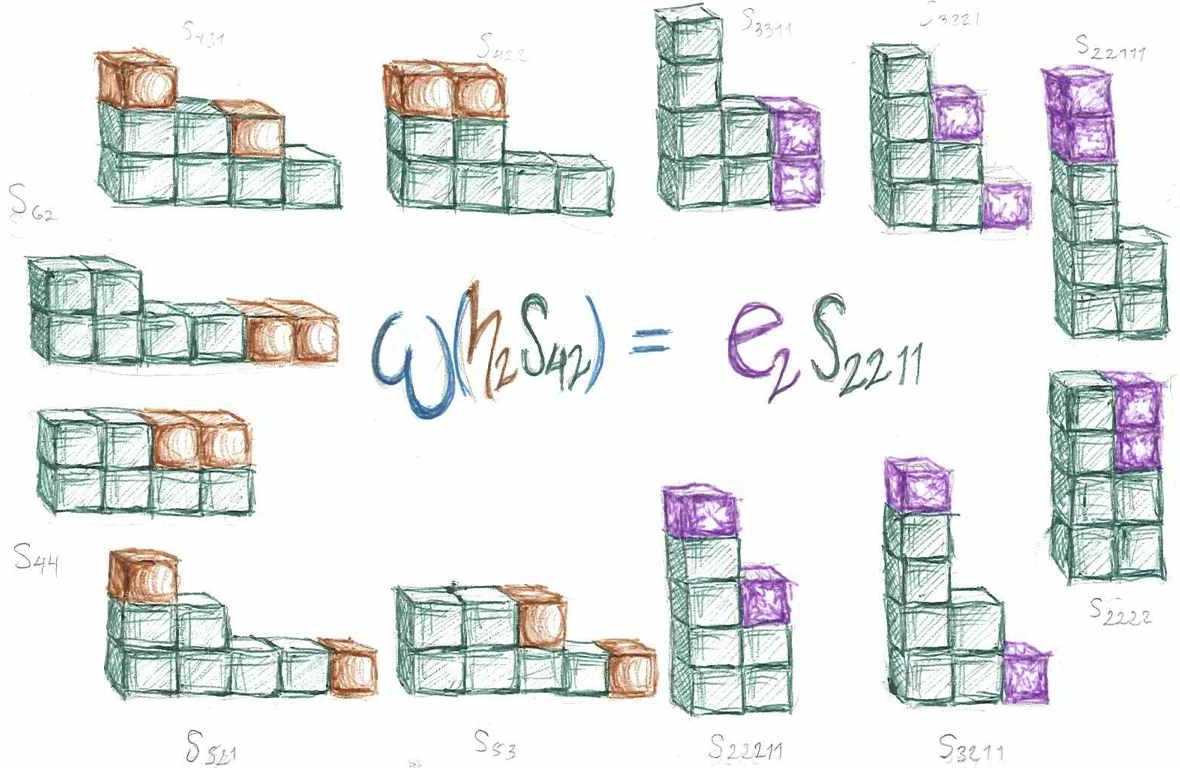Symmetric Functions Video Podcast This is a video textbook introducing the topic of symmetric functions. There is an accompanying tutorial which shows how to compute in this algebra using computer algebra package SAGE.  You might encounter this topic in an advanced undergraduate course or a graduate course in mathematics.  Concepts will be broken down into videos of less than 10 minutes. To understand these videos properly you may need some background in linear algebra (basic concepts such as vector space, dimension, matrix manipulation, linear independence). Exercises and background documentsVideo 1: Definition of the algebra of symmetric functions In this video I introduce the symmetric functions as the algebra of ${\mathbb Q}[h_1, h_2, h_3,\ldots]$ with the degree of $h_r = r$.  Typical questions that come after this video are: Q1. Why define the algebra over ${\mathbb Q}$ (the rational numbers)? A: it could be any field but ${\mathbb Q}$ is general enough and who wants to deal with square roots anyway? Q2. Where are the variables? A: not necessary because these are symmetric functions, not symmetric polynomials.  The relation with symmetric polynomials will appear in a future video.  By defining the symmetric functions this way we avoid taking the inverse limit of polynomials to infinite series.Click to access video PDF of pagesClick to access video PDF of pages Video 2: The definition of the Schur symmetric functions In this video the Schur functions are defined to be determinant of a matrix whose $(i,j)$ entry is $h_{\lambda_i-i+j}$.  This is usually called the Jacobi-Trudi formula for the Schur function (although I failed to mention that during the video).Video 3: The Pieri rule The rule for expanding an $h_r$ times and $s_\lambda$ in the Schur basis is called the Pieri rule. I present it here as a combinatorial rule about how to determine which terms appear by adding a horizontal strip on a partition.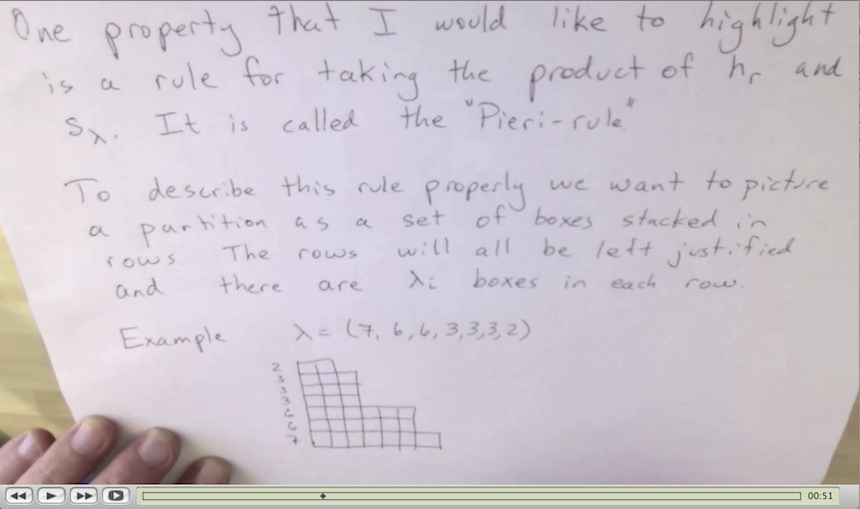Click to access video PDF of pages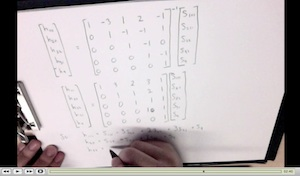Click to access video PDF of pages Video 4: Expanding the h-basis in the Schur basis We can use a couple of methods for expanding an element which is in the h-basis to the Schur basis. Here we describe two. The first is to find how each of the h-basis elements expand in the Schur basis by use of inverting a matrix. The second is to use the triangularity relation between the Schur function and the expansion in the complete basis.Video 5: Expanding the h-basis in the Schur basis using column strict tableaux The coefficient of a Schur function $s_\lambda$ in complete homogeneous basis element $h_\mu$ is equal to the number of column strict tableaux of shape $\lambda$ and content $\mu$. Here we do an example of applying the Pieri rule and a few small examples of how column strict tableaux can be used to give the Schur expansion of a few complete homogeneous elements.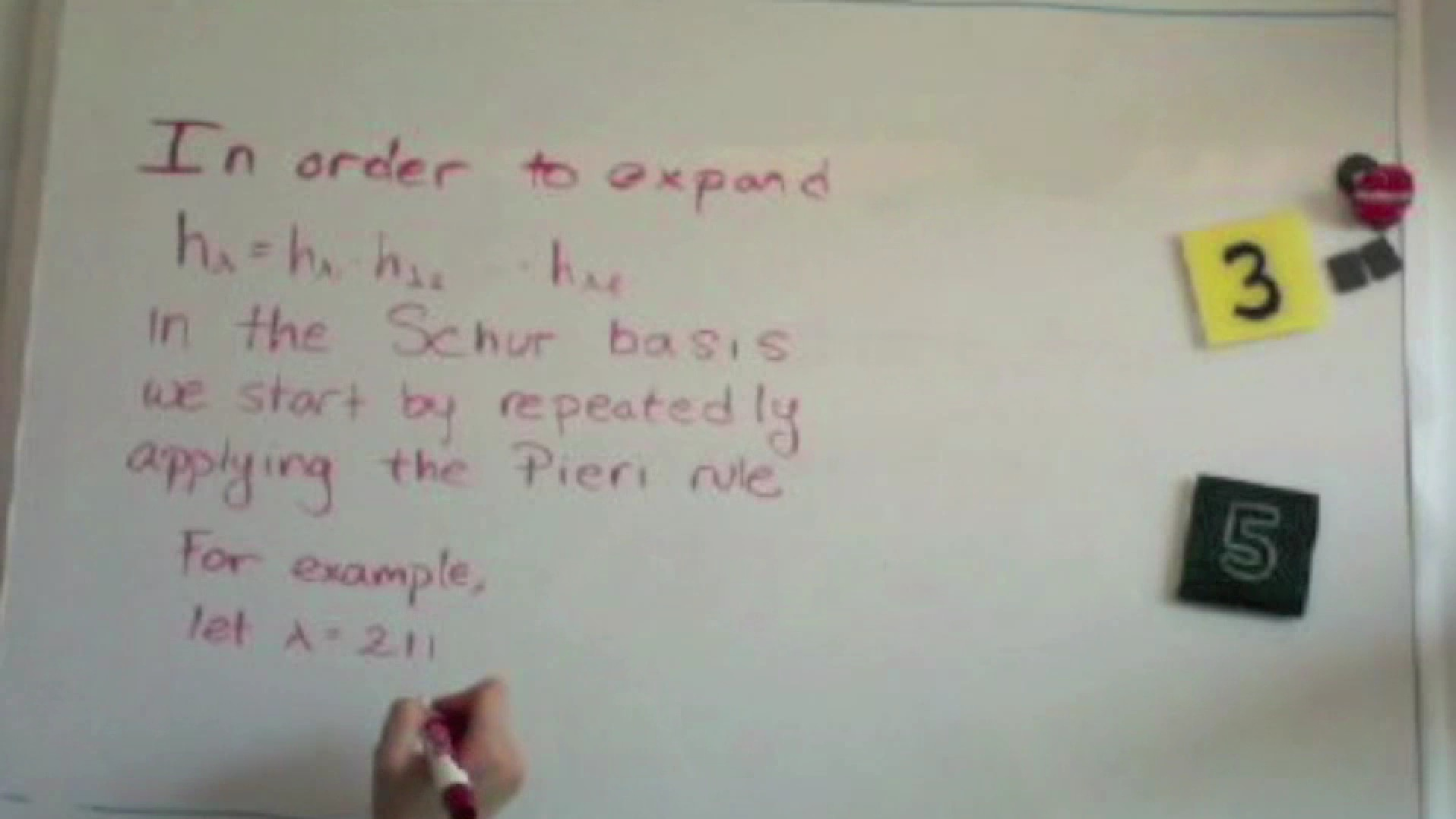Click to access video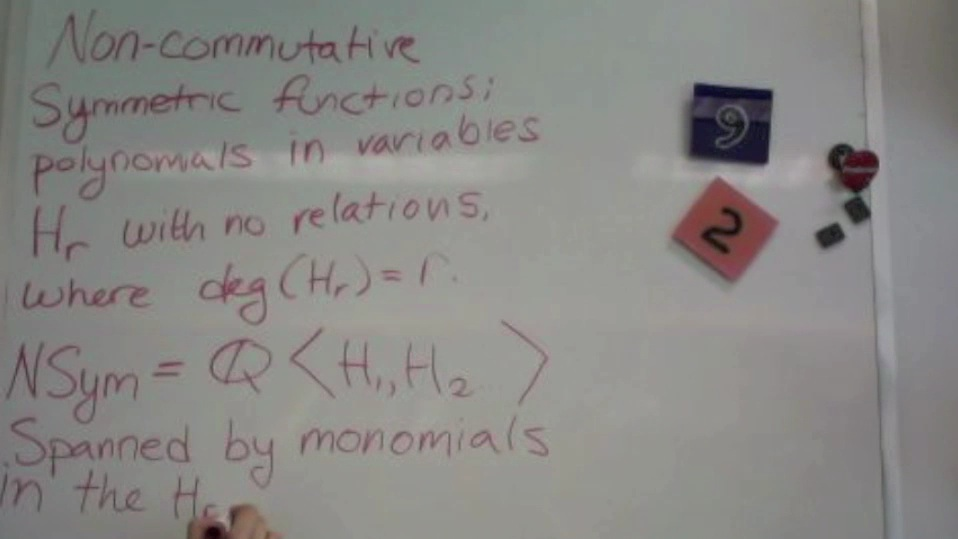Click to access video Video 6: Introduction to the Non-commutative symmetric functions Just as the symmetric functions were defined as the algebra of polynomials in commutative variables $h_r$, we can define the non-commutative symmetric functions as the algebra of non-commutative polynomials in variables $H_r$.Videos by: Chagit Feldman, Jennifer Light, Mike Zabrocki